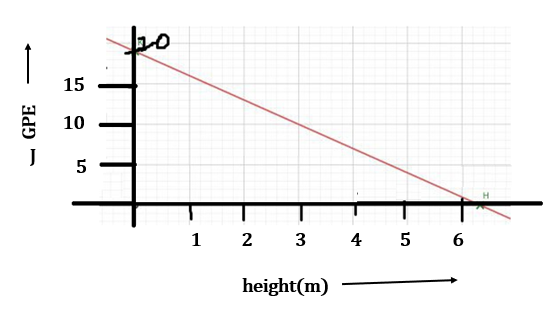Physics
Easy

Question

# What is the value of the gravitational potential energy when the height of the object is4 m

## 5 J7 J7.5 J9 JHint:

## The correct answer is: 7.5 J

### The height of the object is directly proportional to the gravitational potential energy. When the height is 4m, the GPE is the point where the straight line of the graph meets. The GPE is 7.5 J when the height is 4m.#### With Turito Foundation.#### Get an Expert Advice From Turito.